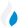•Trade Pi
•Trade
•Exchange
•Trade Pi
•Trade
•Exchange
\$1.12T
Total marketcap
\$87.27B
Total volume
40.20%
BTC dominance
•Trade Pi
•Trade
•Exchange

# matic to IDR Exchange Rate - 1 Polygon in IDR

18,407.507482755
• BTC 0.000052
• ETH 0.0007
Vol [24h]
\$158,281.56

## matic to IDR converter

Exchange Pair Price 24h volume
Indodax MATIC/IDR \$1.22 \$131.2K
Zipmex MATIC/IDR \$1.22 \$24.38K

## MATIC/IDR Exchange Rate Overview

Name Ticker Price % 24h 24h high 24h low 24h volume
Polygon matic \$1.23 4.6445% \$1.25 \$1.17 \$782.24M

Selling 1 Polygon matic you get 18,407.507482755 IDR.

Polygon Dec 27, 2021 had the highest price, at that time trading at its all-time high of \$2.92.

404 days have passed since then, and now the price is 42.16% of the maximum.

Based on the table data, the MATIC vs IDR exchange volume is \$158,281.56.

Using the calculator/converter on this page, you can make the necessary calculations with a pair of Polygon to IDR.

## Q&A

### What is the current MATIC to IDR exchange rate?

Right now, the MATIC/IDR exchange rate is 18,407.507482755.

### What has been the Polygon to IDR trading volume in the last 24 hours?

Relying on the table data, the Polygon to IDR exchange volume is \$158,281.56.

### How can I calculate the amount of IDR? / How do I convert my Polygon to IDR?

You can calculate/convert IDR from MATIC to IDR converter. Also, you can select other currencies from the drop-down list.

## MATIC to IDR Сonversion Table

MATIC IDR
0.4 MATIC = 7,363.002 IDR
0.8 MATIC = 14,726.005 IDR
2 MATIC = 36,815.01 IDR
2 MATIC = 36,815.01 IDR
4 MATIC = 73,630.02 IDR
8 MATIC = 147,260.05 IDR
20 MATIC = 368,150.1 IDR
50 MATIC = 920,375.3 IDR
90 MATIC = 1,656,675.6 IDR
900 MATIC = 16,566,756.7 IDR
9000 MATIC = 165,667,567.3 IDR
90000 MATIC = 1,656,675,673.4 IDR
900000 MATIC = 16,566,756,734.4 IDR